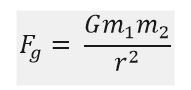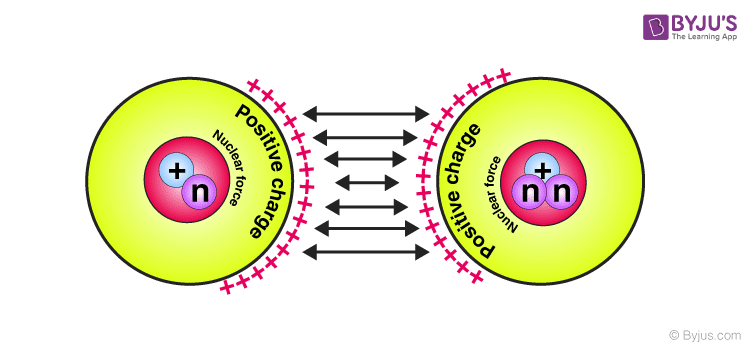# Fundamental Forces In Nature

## What are the Fundamental Forces in Nature?

There are four universal or fundamental forces in nature. Without these forces, all matter in the world will fall apart. Force as such is any pull or push that causes an object to alter its physical state (in terms of motion or deformity). Newton defined a force as anything that causes an object of mass ‘m’ to move with an acceleration ‘a’. Following are the four fundamental forces in nature:

• Gravitational force
• Electromagnetic force
• Strong nuclear force
• Weak nuclear force

A brief overview of the four fundamental forces is given below.

## Gravitational Force

Why is the universe not filled with floating human beings and cows and cars and other such things? Obviously, because the gravitational force of the earth holds us to the planet. Gravitational force is the force responsible for holding planets in their orbits and this is possible only because of their infinitely long-range.

### What is Gravitational Force?

Gravitational force is one of the four fundamental forces of nature. It is the weakest of the four. It is also the attractive force which arises from the gravitational interaction.

According to Newton’s law of gravity, it states that the gravitational force between two bodies is proportional to the product of their masses and inversely proportional to the square of the distance between them.

When considered for massive objects, like the sun, or giant planets, gravitational force is considered to be strong as the masses of these objects are also large. On an atomic level, this force is considered weak.

### Gravitational Force Formula

Gravitational force can be represented as followswhere,

• Fg is the gravitational force
• G is the universal gravitational constant
• m1 and m2 are the masses of the objects in consideration
• r is the distance between the centres of the two objects in consideration

### Gravitational Force of Earth

The gravitational force of the earth is 9.807 m/s2.

### Gravitational Force Examples

• The revolving of the moon around the earth is due to gravitational attraction between them.
• The formation of tides in the ocean is due to the gravitational force acting between the earth and the moon.

Related Articles:

## Electromagnetic Force

Did you know that you have never truly ever touched anything in the world? You are matter, and we know that all matter is made up of atoms. Atoms in turn has a dense nucleus, protons, neutron and electrons. And where are these electrons? They occupy the outermost layer of an atom. Now what is the charge on electrons? They have negative charges.

By their nature, all particles are only attracted to particles that have an opposite charge and repel those with like charges.

Say you feel like holding a pen. The pen is a matter made of atoms filled with nuclei, neutrons, protons and electrons spinning around the nucleus as well. By nature, the electrons that you are made of and the electrons that make up your pen will repel each other. So technically, you never truly touch anything!

These are electromagnetic forces.  Why does a comb get charged when you continuously brush your hair? Why are the subatomic particles held together? Because… Electromagnetic forces!

These have a long-range and the effect of their forces diminishes over distance due to the shielding effect. However, sub-atomically, they have short-range and are considered strong forces but still weaker than strong nuclear forces.

## Strong Nuclear Forces

Out of the four fundamental forces, nuclear forces are the strongest attractive forces. Electromagnetism holds matter together, but there was no explanation on how the nucleus is held together in the atom. If we consider only electromagnetism and gravity, then the nucleus should actually fly off in different directions. But it doesn’t, implying that there exists another force within the nucleus which is stronger than the gravitational force and electromagnetic force. This is where nuclear forces come into play. Strong nuclear forces are responsible for holding the nuclei of atoms together.Same charged protons attract each other due to Nuclear force[/caption]

This is the most dominant force in reactions and is so strong that it binds protons with similar charges with the nucleus. It is mostly attractive in nature but can be repulsive sometimes. It has a very short range of just 1 fm.

## Weak Nuclear Forces

Weak force is the force existing between the elementary particles which are responsible for certain processes to take place at a low probability.

### Weak Nuclear Force Example

Weak nuclear forces are responsible for the radioactive decay, specifically the beta decay neutrino interactions. It has a very short range (less than 1 fm) and this force is, as the name suggests, weak in nature.

### Properties of Weak Force

Following are the properties of weak force:

• Weak nuclear force is the only force which does not follow parity-symmetry.
• It is the only force that does not follow charge-parity symmetry.
• Weak nuclear force is responsible for the change in quark into other forms.
• Carrier particles with significant masses are responsible for the propagation of the weak force.

Q1

### Name the factors affecting the value of acceleration due to gravity.

Following are the factors affecting the value of acceleration due to gravity:

• Height from the earth’s surface
• Latitude of the plane
• Depth
Q2

### What is the distance between the two protons to attract each other?

The distance between the two protons should be 10-15cm so that they attract each other.

Q3

### Name the force responsible for existence of the protons in the nucleus of an atom.

Protons are existing in the nucleus of an atom because of the strong attractive nuclear force which binds them with each other.

Q4

### What is Ampere law?

Ampere law is also known as Ampere circuital law which states that the closed integral of the magnetic field intensity is the same as the current enclosed by it.

## What is Nuclear Force?Test Your Knowledge On What Are The Fundamental Forces In Nature!